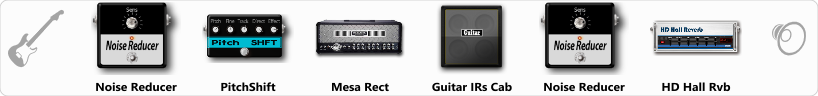# Post Doom Metal 0)))

Discussion in 'ToneLib-GFX presets' started by gian666, Sep 18, 2019.

1. Post Doom Metal 0)))

Preset name: Post Doom Metal 0)))

The name says it all, it is recommended to combine with the Emissary amp which is also free \:v/

[____/Enjoy the Noise\____]

Effects chain:Effect: "Noise Reducer" (Dynamics / Filter), active - "yes"
"Sens" = 50
"Mode" = Hard

Effect: "PitchShift" (Modulation / Sfx), active - "yes"
"Pitch" = 7
"Fine" = 0
"Tracking" = 85
"Direct" = 100
"Effect" = 80

Effect: "Mesa Rect" (Amp simulators), active - "yes"
"Gain" = 88
"Bass" = 80
"Middle" = 58
"Treble" = 53
"Presence" = 42
"Master" = 79
"Output" = 56
"Level (dB)" = 0

Effect: "Guitar IRs Cab" (Cabinets), active - "yes"
"Model" = Orange PPC (4x12")
"Mic Position" = Middle
"Mic Distance" = Near
"Low Cut (Hz)" = 250
"Hi Cut (kHz)" = 4.0
"Mix" = 100
"Level (dB)" = 0

Effect: "Noise Reducer" (Dynamics / Filter), active - "yes"
"Sens" = 100
"Mode" = Hard

Effect: "HD Hall Rvb" (Reverb), active - "yes"
"Time" = 10.0
"PreLPF" = 47
"PreDelay" = 0
"HiDamp" = 27
"LoGain" = -3.3
"Mix" = 49

Note: You will need to download and install the ToneLib-GFX software to use the preset.

File size:
552 bytes
Views:
3,340